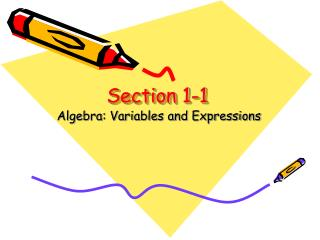Download PresentationSection 1-1

# Section 1-1

Télécharger la présentation## Section 1-1

- - - - - - - - - - - - - - - - - - - - - - - - - - - E N D - - - - - - - - - - - - - - - - - - - - - - - - - - -
##### Presentation Transcript

1. Section 1-1 Algebra: Variables and Expressions

2. Why study algebra?

3. Variable • Variable: Symbols are used to represent unspecified numbers or values • “vary”; it means that the value can vary, not fixed. • a, b, z, e, f • Ex 1: Let t be the temperature of each day in a week. Is temperature a variable? • Ex. 2: Is 5 a variable? • Ex. 3: Let ϐ be the weight of a student in class. Is the weight a variable?

4. Algebraic Expression • “expression” means what? • consists of one or more numbers and variables and with one or more arithmetic operations. • string of numbers and operators that can be placed on one side of an equation like 2+3. • Ex. 1: Is x + 2 =3 an algebraic expression? • Ex.2: Is 3x-7 an algebraic expression? • Ex.3: Is 25-10 an algebraic expression? • Ex. 3: Come up with your own algebraic expression.

5. Factor • xy x(y) (x)y (x)(y) • Algebraic expression consist a raised dot or parentheses. This means multiplication. • Factor is quantities being multiplied • Ex 1: Is 5x a factor? • Ex 2: Is (y+3) a factor? Is y a factor?; x(y+3) = xy+3x • Is there anything unusual on the right side of the equation? There is a ‘common factor.’ • Is xy + 3x equal to x(y+3)? Why? It is commutative. • 2 – 3 =?= 3-2 What do we think of when we hear the term ‘product’? Multiplication! The result is called the product.

6. Exponent • Exponent (or powers): repeated multiplication • xn= x· x· x· x· x·… x • variable x is the base and n is the exponent. • Ex: Evaluate expression 33. (3 · 3· 3 = 27) • What is the base number? What is the exponent? • How do we read 33 ? “3 to the power of 3”, “3 to the third power,” or “3 cubed” • Ex: Evaluate expression 22. (2 · 2= 4) • What is the base number? What is the exponent? • How do we read 22 ? “2 to the power of 2”, “2 to the second power”

7. Writing Algebraic Expression • Write each expression algebraically. • Ex: the product of 2 and a to the third power; 2a3 -Ex: the sum of 11 and x to the third power; 11+x3

8. Writing Algebraic Expression • Write an algebraic expression for each verbal expression. • Ex: five more than a number n • 5+n • Ex:7 less than the product of 4 and a number x • 7-4x • Ex: one third of the size of the original area a • 1/3a or a/3

9. Writing Verbal Expression • Write a verbal expression for each algebraic expression • Ex: 11+x3 • the sum of 11 and x to the third power • Ex: c2 +x3 • the sum of c squared and 21 times d

10. Conclusion • Conclusion: What have we learned today? • Variables • Algebraic expression • Factors and product • Exponent, power, base • Translating algebraic expression to verbal expression and vice-versa • What else? • Homework: • * Review notes • * Read p. 8 “Translating from English to Algebra”